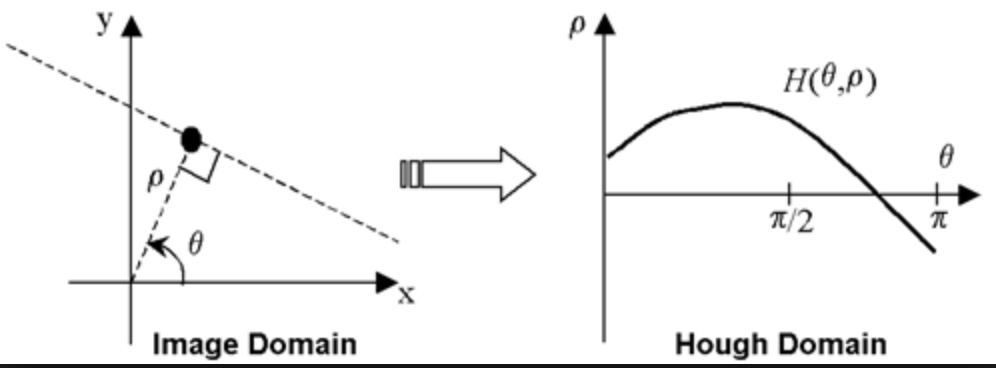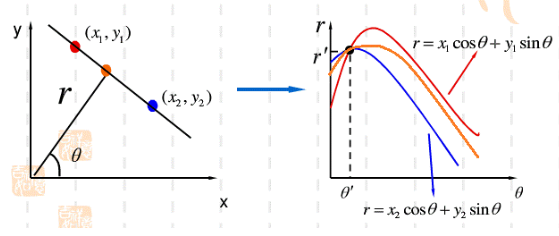# Image Processing-Image Segmentation

Posted by GwanSiu on December 27, 2017

In this article, some basic segmentation techniques are introduced: (1). Hough Transform; (2). Otsu’s Segmentation; (3).Interactive Image Segmentation; (4). Graph Cuts; (5).Mumford Shah; (6). Active Contours Algorithm;

## 1. Hough Transform

Hough transform is a widely used shape detection techniques in computer vision and image processing. Hough transform aims to find imperfect instances of objects of within a certain class of shapes by a voting procedure. Essentially, hough transform transform points in the original space to parametric sapce, in which there is a accumulator to calculate the intersection of line, and the maximum points in the parametric space is the shape represented in the original space by multicollinearity.For example, hough transform transform points of a line in cartesian coordinate system to polar coordinate system. Why polar coordinate system? The line is represented in cartesian coordinate system: $y=kx+b$, and the parameters are $k$ and $b$, where $k\in [-\infty,+\infty],b\in[-\infty,+\infty]$, it means that when the line with $k\approx \infty$ cannot be represented in the cartesian coordinate system. For each point in the original space, we draw all line across the point in the parametric sapce. We can see that each $\theta$ in parametric space represent a line in the original space. Therefore, the intersection in the parametric space is the line across all points in the original spacel.## 2. Otsu’s Algorithm

Otsu method is a popular method in image segmentation, which aims to automatically perform clustering-based image thresholding, or, the reduction of a graylevel image to a binary image.

Otsu algorithm assumes that image contains two classes of pixels following bi-modal histogram(foreground pixel and background pixels), it then calculates the optimum threshold separating the two classes so that inter-variance is maximized. Consequently, otsu’s algorithm is roughly a one-dimensional, discrete analog of Fisher’s discriminant analysis.

Otsu algorithm exhaustively search for the threshlod that minimizes the inter-class variances, the objective function is defined as a weighted sum of variances of the two classes:

where weights $\omega_{0}$ and $\omega_{1}$ are the probability of the two classes separated by a threshold $t$, and $\sigma_{0}$ and $\sigma_{1}$ are variances of these two classes. The class probability $\omega_{0}$ and $\omega_{1}$ are computed from the $L$ bins of the histogram:

Otsu shows that minimizing the intra-class variance is equivalent to maximize inter-class variance:

This equation shows the relationship of class probability $\omega$ and class means $\mu$.

while the class mean $\mu_{0}(t),\mu_{1}(t),\mu_{T}$ is:

The following relations can be easily verified:

Algorithm

1. Compute the histogram and probabilities of each intensity level
2. Set up initial $\omega_{i}(0)$ and $\mu_{i}(0)$
3. Step through all possible threshold $t=1,…,$ maximum intensity
1. Update $\omega_{i}$ and $\mu_{i}$
2. Compute $\sigma_{b}^{2}(t)$
4. Desired threshold corresponds to the maximum $\sigma_{b}^{2}(t)$

## 5. Active Contours Algorithm